• 针对GF空间多项式函数,在范数限定条件下利用多核学习方法优化多项式权系数,实现多项式函数的优化。实验结果表明,算法优化得到的多项式函数其分类性能优于常用的单核函数,与多核方法相当,并在分类中取得良好...
• 1．统计学习是关于计算机基于数据...3．本书主要讨论监督学习，监督学习可以概括如下：从给定有限的训练数据出发， 假设数据是独立同分布的，而且假设模型属于某个假设空间，应用某一评价准则，从假设空间中选取一个...
1．统计学习是关于计算机基于数据构建概率统计模型并运用模型对数据进行分析与预测的一门学科。统计学习包括监督学习、非监督学习、半监督学习和强化学习。
2．统计学习方法三要素——模型、策略、算法，对理解统计学习方法起到提纲挈领的作用。
3．本书主要讨论监督学习，监督学习可以概括如下：从给定有限的训练数据出发， 假设数据是独立同分布的，而且假设模型属于某个假设空间，应用某一评价准则，从假设空间中选取一个最优的模型，使它对已给训练数据及未知测试数据在给定评价标准意义下有最准确的预测。
4．统计学习中，进行模型选择或者说提高学习的泛化能力是一个重要问题。如果只考虑减少训练误差，就可能产生过拟合现象。模型选择的方法有正则化与交叉验证。学习方法泛化能力的分析是统计学习理论研究的重要课题。
5．分类问题、标注问题和回归问题都是监督学习的重要问题。本书中介绍的统计学习方法包括感知机、K近邻法、朴素贝叶斯法、决策树、逻辑斯谛回归与最大熵模型、支持向量机、提升方法、EM 算法、隐马尔可夫模型和条件随机场。这些方法是主要的分类、标注以及回归方法。它们又可以归类为生成方法与判别方法。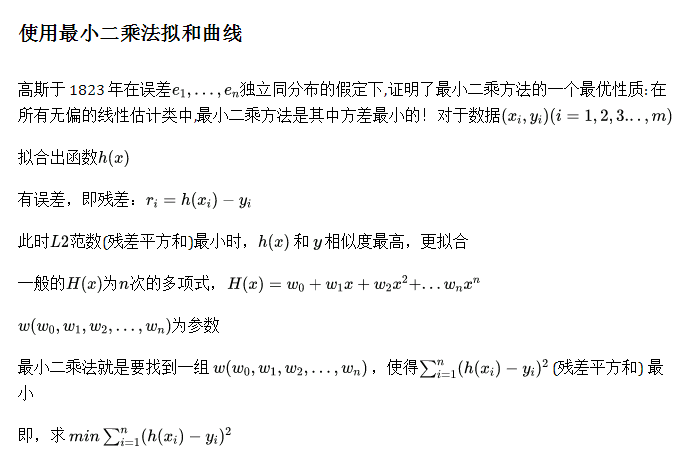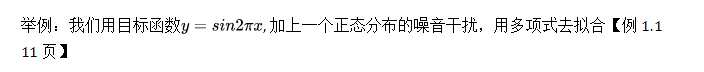import numpy as np
import matplotlib.pyplot as plt
from scipy.optimize import leastsq

# 我们要拟合的目标函数
def real_func(x):
return np.sin(2*np.pi*x)

# 我们自己定义的多项式函数
def fit_func(p, x):
f = np.poly1d(p)  # np.poly1d([2,3,5,7])返回的是函数，2x3 + 3x2 + 5x + 7
ret = f(x)
return ret

# 计算残差
def residuals_func(p, x, y):
ret = fit_func(p, x) - y
return ret

def fitting(M=0):
"""
M    为 多项式的次数
"""
# 随机初始化多项式参数
p_init = np.random.rand(M + 1)  # 返回M+1个随机数作为多项式的参数
# 最小二乘法:具体函数的用法参见我的博客：残差函数，残差函数中参数一，其他的参数
p_lsq = leastsq(residuals_func, p_init, args=(x, y))
# 求解出来的是多项式当中的参数，就是最小二乘法中拟合曲线的系数
# print('Fitting Parameters:', p_lsq)
return p_lsq

# 书中10个点,对y加上了正态分布的残差
x = np.linspace(0, 1, 10)
y_old = real_func(x)
y = [np.random.normal(0, 0.1) + yi for yi in y_old]

x_real = np.linspace(0, 1, 1000)
y_real = real_func(x_real)

plt.plot(x_real, y_real, label="real")
plt.plot(x, y, 'bo', label='point')
#  fiitting函数中args=（x， y）是条用的是上面定义的10个点的全局变量x,y
plt.plot(x_real, fit_func(fitting(9), x_real), label="fitted curve")
plt.legend()
plt.show()

M=0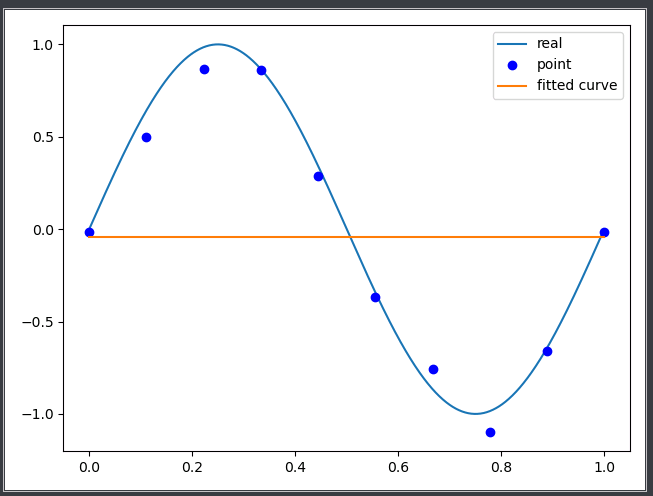M=1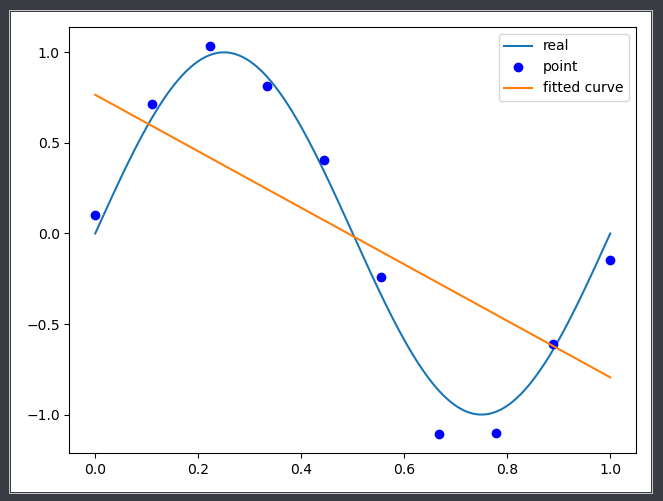M=3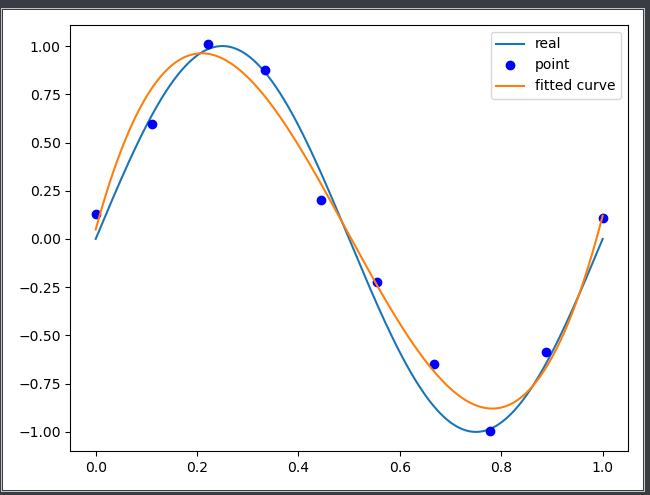M=9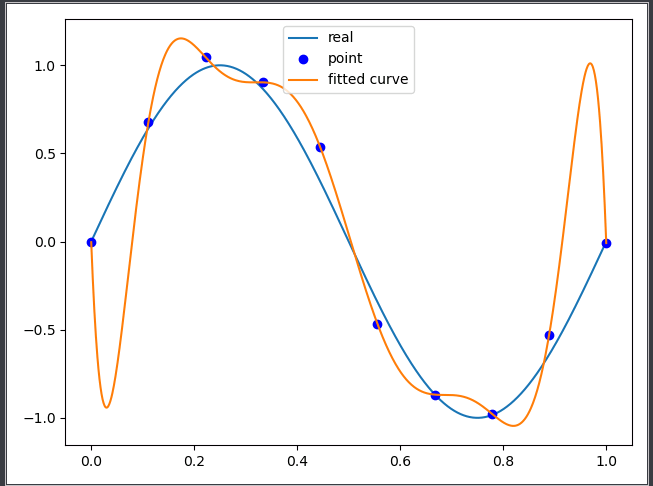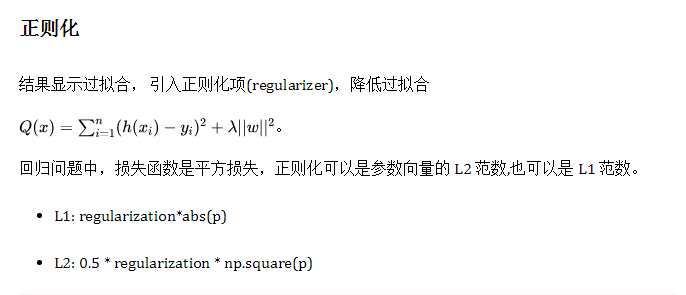W是参数，就是最小二乘法求得到的系数
lambda是regularization，是自定义的系数。
import numpy as np
import matplotlib.pyplot as plt
from scipy.optimize import leastsq

# 我们要拟合的目标函数
def real_func(x):
return np.sin(2*np.pi*x)

# 我们自己定义的多项式函数
def fit_func(p, x):
f = np.poly1d(p)  # np.poly1d([2,3,5,7])返回的是函数，2x3 + 3x2 + 5x + 7
ret = f(x)
return ret

# 计算残差
def residuals_func(p, x, y):
ret = fit_func(p, x) - y
return ret

# 返回残差和正则项
def residuals_func_regularization(p, x, y):
ret = fit_func(p, x) - y
ret = np.append(ret,
np.sqrt(0.5 * regularization * np.square(p)))  # L2范数作为正则化项
return ret

def fitting(M=0):
"""
M    为 多项式的次数
"""
# 随机初始化多项式参数
p_init = np.random.rand(M + 1)  # 返回M+1个随机数作为多项式的参数
# 最小二乘法:具体函数的用法参见我的博客：残差函数，残差函数中参数一，其他的参数
p_lsq = leastsq(residuals_func, p_init, args=(x, y))
# 求解出来的是多项式当中的参数，就是最小二乘法中拟合曲线的系数
# print('Fitting Parameters:', p_lsq)
return p_lsq

# 书中10个点,对y加上了正态分布的残差
x = np.linspace(0, 1, 10)
y_old = real_func(x)
y = [np.random.normal(0, 0.1) + yi for yi in y_old]

x_real = np.linspace(0, 1, 1000)
y_real = real_func(x_real)

# # 画出10个散点，sin图像，和拟合的曲线
# plt.plot(x_real, y_real, label="real")
# plt.plot(x, y, 'bo', label='point')
# plt.plot(x_real, fit_func(fitting(9), x_real), label="fitted curve")
# plt.legend()
# plt.show()

# 画出添加正则项的曲线
regularization = 0.0001
p_init = np.random.rand(9 + 1)
p_lsq_regularization = leastsq(
residuals_func_regularization, p_init, args=(x, y))

# 画出原sin图像，不加正则项的图像，加上正则项的图像，10个点的散点图
# 不加正则项和加上正则项都是9次方，10个系数
plt.plot(x_real, real_func(x_real), label='real')
plt.plot(x_real, fit_func(fitting(9), x_real), label='fitted curve')
plt.plot(
x_real,
fit_func(p_lsq_regularization, x_real),
label='regularization')
plt.plot(x, y, 'bo', label='noise')
plt.legend()
plt.show()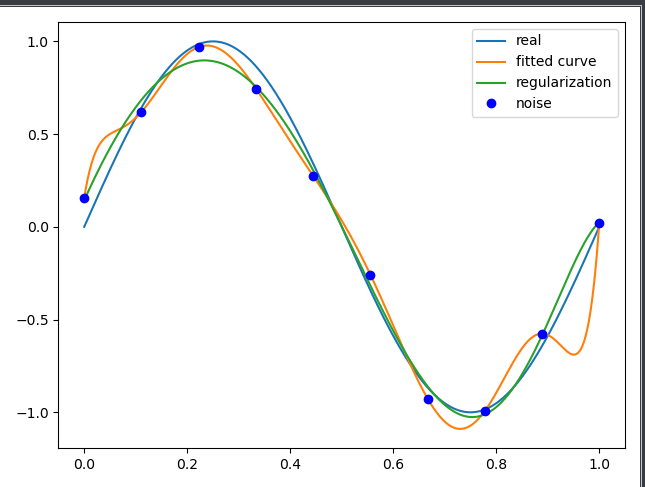展开全文python 机器学习
•研究论文
• 本文可作为线性代数实现线性回归的下篇,先简单回顾一下，线性代数实现线性回归中介绍了子空间的概念，把子空间...本文将拓展子空间的概念，空间的元素是函数称之为函数空间，这个空间里面有我们熟悉的各种函数以及这...
  本文可作为线性代数实现线性回归的下篇,先简单回顾一下，线性代数实现线性回归中介绍了子空间的概念，把子空间想象成一个超平面，子空间中任意一个向量都可以用子空间的基线性组成，线性回归原理是已知一个超平面和超平面外的一个向量，该向量与在超平面上的投影距离最短，或者说误差最小，在得到这个投影的同时就知道了未知参数，未知参数是投影在子空间基上的坐标。

上篇中介绍的空间是一个由向量组成的空间，向量中元素是实数。 本文将拓展子空间的概念，空间的元素是函数称之为函数空间，这个空间里面有我们熟悉的各种函数以及这些函数的线性组合。函数空间里的子空间是一系列性质相似的函数集合，比如三角函数可以组成一个子空间，由数学分析知道利用三角函数可以实现傅里叶变换，将其他函数表示为三角函数线性组合成的级数形式。本文中选取的空间是一个多项式空间，即其空间里基是x0,x1,x2,x3,...xn...等多项式，需要将其他函数投影到这个子空间里，其运用的知识还是来自于线性代数。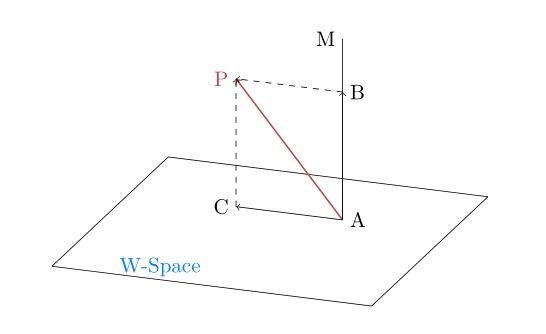使用上图来说明函数空间，AP代表了函数空间里的一个函数，而下面的平面代表了多项式子空间，AP在多项式空间里的投影AC就是与AP误差最小的多项式，AC是x0,x1,x2,x3,...xn等多项式的线性组合，我们目标就是求出这个线性组合表达式中每个基前面的系数(坐标)。

一、一元多项式逼近任意一元连续函数

这里结合一段python代码说明，这段代码的功能是利用多项式逼近函数f(x)=sin(x)-3cos(x)。

import numpy as np

import math

from sympy import *

from scipy.integrate import tplquad, dblquad, quad

from scipy.linalg import *

import matplotlib.pyplot as plt

plt.rcParams['font.sans-serif'] = ['SimHei']

plt.rcParams['axes.unicode_minus'] = False

NSplit_Num = 10000

class polynomialAppro(object):

def __init__(self, split=1000):

self.splitnum = split

pass

def genfunction(self):

ar = []

t = np.linspace(-math.pi, math.pi, self.splitnum)

for x in t:
ar.append(math.sin(x) - 3 * math.cos(x))

sx = np.expand_dims(np.asarray(ar), 0)

return sx

def genpolynomialdata(self):

ar = []

t = np.linspace(-math.pi, math.pi, self.splitnum)

for x in t:
ar.append([1, x, x ** 2, x ** 3, x ** 4, x ** 5])

polynomialdata = np.asarray(ar)

return polynomialdata

if __name__ == '__main__':
appro = polynomialAppro(NSplit_Num)

sx = appro.genfunction()  # 1.1

b = sx.T  # 1.2

A = appro.genpolynomialdata()  # 1.3

ATAminus = np.linalg.inv(np.dot(A.T, A))  # 1.4

coordinate = np.round(np.dot(ATAminus, np.dot(A.T, b)), 4)  # 1.5

# 打印图像验证

x = np.linspace(-math.pi, math.pi, NSplit_Num)

y_x = coordinate+ coordinate * x + coordinate * (x ** 2)+  coordinate * (x ** 3) + coordinate * (
x ** 4) + coordinate * (x ** 5)

plt.figure()

plt.subplot(121)

plt.title('原函数图像')

plt.plot(x, b[..., 0])

plt.subplot(122)

plt.title('多项式逼近的函数图像')

plt.plot(x, y_x)

plt.show()

来看下模拟的效果图: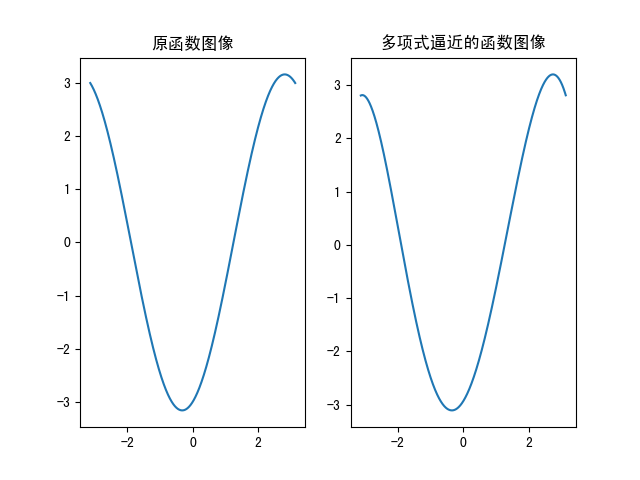可以看到利用线性回归求出的多项式函数可以较好的逼近目标函数，下面就代码中标红色部分说明：

#1.1 sx=appro.genfunction()

这里是得到目标函数f(x)=sin(x)-3cos(x)在-π ，π之间的数据，将其离散化变成计算机可以处理的向量形式，这里是为了说明采用了显式函数结构，真实的应用中可以是采集而来样本数据。

#1.2 b=sx.T

为了后期处理方便，将其处理成10000*1的向量形式。

#1.3 A=appro.genpolynomialdata()

选取x0,x1,x2,x3,x4,x5作为子空间的基，并将其向量化，这里将得到一个10000*6的矩阵，此时A就是在介绍线性回归问题时的子空间。这里将函数空间元素赋值，使一个函数空间问题变为一个线性代数问题，这种离散化数据手段在计算机处理中会经常使用。

#1.4、1.5

ATAminus=np.linalg.inv( np.dot(A.T,A))

coordinate= np.round(np.dot(ATAminus,np.dot(A.T,b)),4)

经过前面离散化处理，此时问题就变成利用线性空间求解线性回归问题，#1.4、1.5 是求出函数f(x)=sin(x)-3cos(x)在多项式子空间上的投影，这个处理过程 在之前已经讨论过，这里再复盘一遍。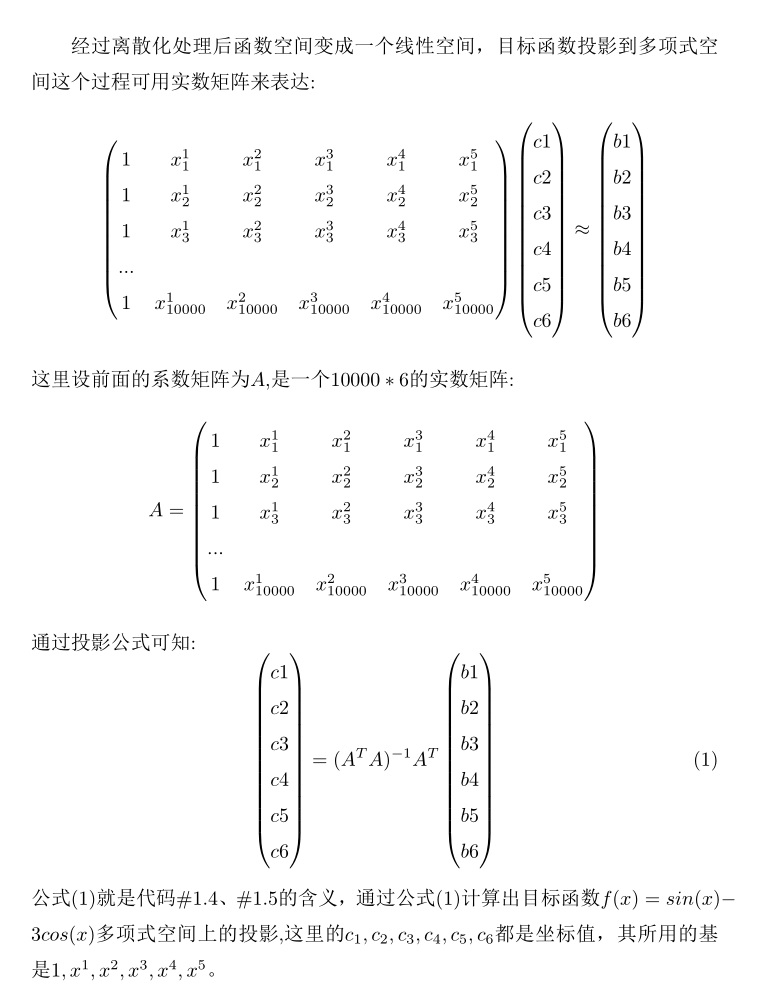二、 二元多项式逼近任意连续二元连续函数

二元乃至多元多项式逼近任意连续函数与一元函数类似，都是最终转化为实数矩阵形式，然后利用公式(1)求解系数，只不过在构造多项式形式上略有不同，掌握二元多项式逼近问题即可推广至任意元函数的情形，这里还是先展示一段python代码。

from matplotlib import pyplot as plt

from mpl_toolkits.mplot3d import Axes3D

import numpy as np

import  math

fig = plt.figure()  #定义新的三维坐标轴

ax3 =  fig.add_subplot(211,projection='3d')

ax2 =  fig.add_subplot(212,projection='3d')

#定义三维数据

NSTP=500

nstart=-math.pi

nend= math.pi

Lens= nend-nstart

xx = np.arange(nstart,nend,Lens/NSTP)

yy = np.arange(nstart,nend,Lens/NSTP)

X, Y = np.meshgrid(xx, yy)

Z =  np.sin(X)-np.cos(Y)

ar=[]

tempa=[]

for x in xx:

for y in yy:

ar.append(   math.sin(x)-math.cos(y))

tempa.append([1,(y**1) ,(y**2) ,(y**3)  , (x**1) ,(y**1)*(x**1),(y**2)*(x**1) ,(x**1)*(y**3),(x**2)*(y**0),(x**2)*(y**1),(x**2)*(y**2),(x**2)*(y**3)

,(x**3)*(y**0),(x**3)*(y**1),(x**3)*(y**2),(x**3)*(y**3)])#2.1

pass

A=np.array(tempa)

b=np.array(ar).reshape([NSTP**2,1])

aminus = np.linalg.inv(np.dot(A.T, A))

temp = np.dot(A.T, b)

c = np.dot(aminus, temp)

#作图验证

Z2 = c[0,0]*1+ c[1,0]*Y + c[2,0]*(Y**2) +  c[3,0]*(Y**3) + c[4,0]*(X**1) + c[5,0]*(X**1)*(Y**1) + c[6,0]*(X**1)*(Y**2) + c[7,0]*(X**1)*(Y**3) + c[8,0]*(X**2) + c[9,0]*(X**2)*(Y**1)+ c[10,0]*(X**2)*(Y**2) + c[11,0]*(X**2)*(Y**3) + c[12,0]*(X**3) + c[13,0]*(X**3)*(Y**1) + c[14,0]*(X**3)*(Y**2)+  c[15,0]*(X**3)*(Y**3)

ax3.plot_surface(X,Y,Z,cmap='Blues')

ax2.plot_surface(X,Y,Z2,cmap='Greens')

#ax3.contour(X,Y,Z, zdim='z',offset=-2，cmap='rainbow)   #等高线图，要设置offset，为Z的最小值

plt.show()

效果图如下，上面是目标函数f(x,y)=sin(x)-cos(y)图像，下面是多项式拟合的函数图像: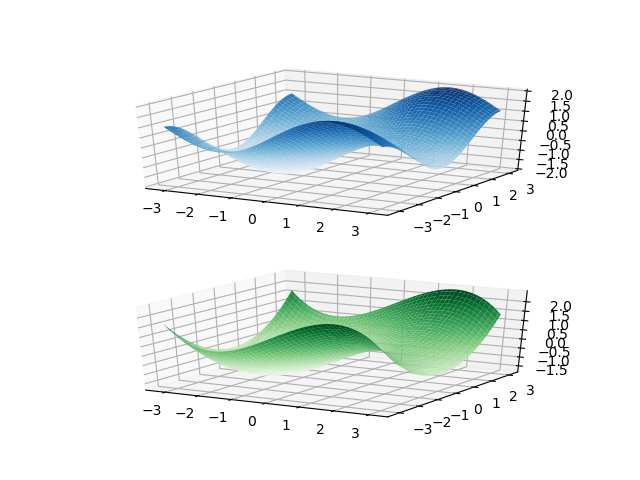可以发现多元函数求解系数与一元函数一模一样，可以完全使用一元函数时所使用的代码，如上面的红色区域，唯一不同的是这里是二元函数，本例选择多项式最高为6次，即X,Y最高次数为3次，可以选择更高次数以加强精确度。这里需要关心的问题是次数定下来后，基的组合有哪些，既然说到组合，首先想到应该是组合数学。

余下文章链接 多项式逼近

展开全文数据挖掘 机器学习 深度学习
• 本文研究了Bernstein-Durrmeyer代数多项式倒数对非负连续函数在Orlicz空间中的逼近问题．利用光滑模和K-泛函等工具，获得了收敛速度的估计，所得的结果比Lp空间内的相应结果具有拓展的意义．
• 用循环链表作稀疏多项式的存储结构,编写求导函数的算法,利用原多项式的结点空间存放其异函数,同时释放所有无用节点. void Qiu_Dao_LinkedPoly(LInklist &L) //对有头结点循环链表结构存储的稀疏多项式L求导 { p=...
用循环链表作稀疏多项式的存储结构,编写求导函数的算法,利用原多项式的结点空间存放其异函数,同时释放所有无用节点.
void Qiu_Dao_LinkedPoly(LInklist &L)
//对有头结点循环链表结构存储的稀疏多项式L求导
{
p=L->next;
if(!p->data.exp)
{
L->next=p->next;p=p->next;//跳过常数项
}
while(p!=L)
{
p->data.coef*=p->data.exp--;//对每一项求导
p=p->next;
}
}//QiuDao_Linkedpoly

展开全文• 三角插值matlab代码数值分析方法 概述 这是我为数值分析类编写的MATLAB函数的集合： 多项式求根方法 函数插值方法 三角曲面 要求 为了运行此代码，您需要具有MATLAB的工作副本以及使用它的一些以前的经验。
• 一，有序齐次单项式空间2阶有序...我们观察二阶有序齐次单项式，在H中做内积有： 我们定义函数： 由此我们推算到d阶的有序齐次单项式： 二，有序单项式空间类似的可以推导出，二阶有序单向式空间： 类似的推导到...
一，有序齐次单项式空间2阶有序齐次单项式：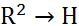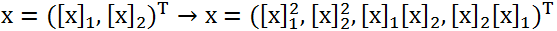由此推广，可以得到d阶的有序齐次单项式：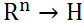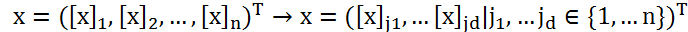由上式可知，如果n和d数据过大时，内积的运算量将会非常大。我们观察二阶有序齐次单项式，在H中做内积有：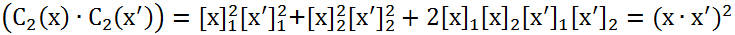我们定义函数：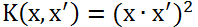由此我们推算到d阶的有序齐次单项式：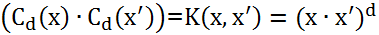二，有序单项式空间类似的可以推导出，二阶有序单向式空间：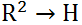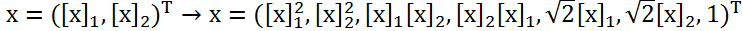类似的推导到多阶：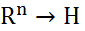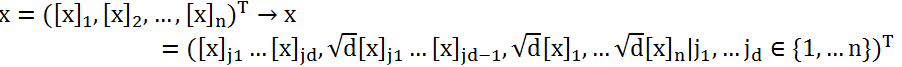类似推定义函数：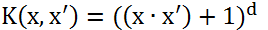推算到d阶的有序单项式：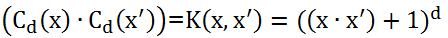三，无序单项式空间在上述有序的单项式中，我们把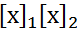和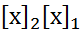看做不同的分量而区分，而实际上我们可以把它看做是无序的，相等的，将映射调整为：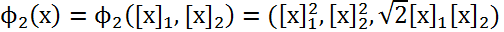仍然可以得出：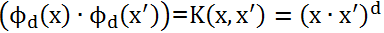四，Hilbert空间与多项式核函数由前面可知支持向量分类机只与内积有关系，而与映射到的Hilbert空间并无直接联系，所以以后我们只关系内积。我们把此函数统称为多项式核：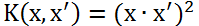和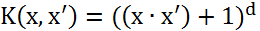例子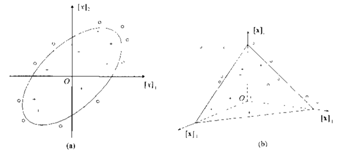假设一个椭圆可以对类进行划分（如图（a））：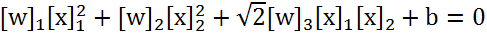引入非线性映射：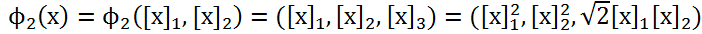获得三维空间，使用支持向量机分划超平面（如图(b)）: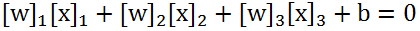展开全文• Status是函数的类型,其值是函数结果状态代码，如OK等 / typedef int Boolean; / Boolean是布尔类型,其值是TRUE或FALSE */ typedef int ElemType; typedef struct{ int coef; int exp; }PolyTerm; typedef ...
• 为了用一种模型实现逼近与插值的统一，在多项式函数空间上构造了含两组参数的混合函数，并由之定义了基于四点分段的多项式曲线和相应的张量积曲面。当参数取特殊值时，新曲线曲面成为三次均匀B样条曲线曲面。除了...B样条曲线曲面
• 将Malliavin在半平面关于一元解析函数唯一性的定理推广到多重复零点情形,并推广到多元情形,利用所得结果研究了多元复指数函数系对多元实函数的加权逼近及其闭包的描述。
• 运用凸函数的Jensen不等式、K -泛函和 Hardy- Littlewood极大函数等工具,研究了 Bernstein-Durrmeyer多项式在 Orlicz空间内的逼近性质,建立了逼近正逆定理.
• ## 基函数与函数空间

千次阅读 2018-09-05 16:10:57
引言 在学习线性回归模型的时候就会遇到基函数，可能我们会遇到多项式基函数、高斯基函数、sigmoid基函数，当然在高等数学和信号...不过还是对基函数与函数空间的关系、基函数的深层认识模棱两可。我希望能通过这...
• 在一个月之前我已经对有限元方法数学理论之分片一次多项式空间逼近理论进行了讲解，由于中间有点事情所以对二次多项式情况没有进行解说，下面开始正文. 其实思想跟一次多项式的情况一样，只不过这里在一个小区间上...
• 对于分片一次多项式空间内的基函数该是怎样的呢？下面就来讲述基函数构造问题。 对于区间[x1,xn+1]上进行剖分（均匀或者非均匀剖分），然后构造如下图的分段函数： 其中Ti(x)为分段基函数，分别在区间[xi-1,xi]和...
• 本文考虑了函数f∈Lp [0,2π],1≤p≤∞的特定的修正插值多项式,并给出了插值多项式函数f 的逼近速度的估计。本文的估计改进了Metelichenko 最近的结果。
• 讨论了LBMa空间上的单调函数用单调多项式的逼近问题,构造了两个线性且保持单调的算子Sn (f,x )和Ln (f,x ),证明了它们在LBMa空间上有界,并且与f的误差可以用二阶带权连续模控制.
• Bézier曲线曲面是张量积形式的，为了进一步研究非多项式空间中的T-Bézier基，完善其关于三角域部分的理论，将4阶T-Bézier基推广到三角域上，构造了满足非负性、规范性、对称性、边界性质和线性无关性的基函数，...
• 讨论了Orlicz空间上的单调函数用单调多项式的逼近问题，构造了两个线性且保持单调的算子-Sn(f，x)和-Ln(f，x)，证明它们在Drlicz空间上有界，且它们和f的误差可用的二阶带权连续模控制。
• 时间限制：40s 空间限制：256MB 题目描述 刚刚解决完电力网络的问题, 阿狸又被领导的任务给难住了. 刚才说过, 阿狸的国家有n个城市, 现在国家需要在某些城市对之间建立一些贸易路线, 使得整个...
• 【机器学习中的数学】基函数与函数空间引言 在学习线性回归模型的时候就会遇到基函数，可能我们会遇到多项式基函数、高斯基函数、sigmoid基函数，当然在高等数学和信号系统中还经常会碰到傅里叶基。有时候，不禁要问...
• 【机器学习中的数学】基函数与函数空间JasonDing关注2015.11.16 21:23:32字数 2,167阅读 9,981引言 在学习线性回归模型的时候就会遇到基函数，可能我们会遇到多项式基函数、高斯基函数、sigmoid基函数，当然在高等...
• 在状态空间方程中引入输入和状态的多项式函数, 以此多项式函数表示非线性因素. 为了辨识多项式非线性系统中的各系统矩阵, 对于矢量化各系统矩阵组成的未知参数矢量, 分别在无约束和有约束条件下采用两并行分布算法...
• 空间存放其导函数多项式），同时释放所有无 用（被删）结点。 实现下列函数： void Difference(LinkedPoly &pa); /* 稀疏多项式 pa 以循环链表作存储结构， */ /* 将此链表修改成它的导函数，并释放无用...c语言 数据结构 算法
• Ⅰ.多元周期函数的非整数次积分设f(P)是在整个m维空间Ω上连续的函数,并且假定积分......
• 并介绍了其他的函数空间的基♂ 比如泰勒展开 就是多项式基 这些文章给了我们新思路：可以从线性变换去考虑这些东西。 正交化过程的理解：之前一直是死机公式。。。 　内积：某一向量在另一向量上投影 与 另一...
• 显然的做法是求出斯特林数，但没有什么优化空间。 　考虑一种暴力dp，即设f[i]为i块积木的所有方案层数之和，g[i]为i块积木的方案数。转移时枚举第一层是哪些积木，于是有f[i]=g[i]+ΣC(i,j)·f[i-j]，g[i]=ΣC(i,j......